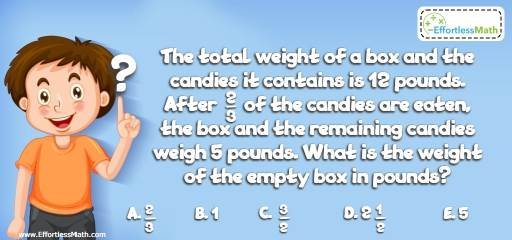# Geometry Puzzle – Challenge 71

This is a fun and engaging math puzzle to challenge even the smartest students and help them develop logic skills.## Challenge:

The total weight of a box and the candies it contains is 12 pounds. After $$\frac{2}{3}$$ of the candies are eaten, the box and the remaining candies weigh 5 pounds. What is the weight of the empty box in pounds?

A- $$\frac{2}{3}$$

B- 1

C- $$\frac{3}{2}$$

D- $$2\frac{1}{2}$$

E- 5

### The Absolute Best Book to challenge your Smart Student!

Let “B” be the weight of the box and “C” be the weight of the candies. So:
B + C = 12
After $$\frac{2}{3}$$ of the candies are eaten, the box and the remaining candies weigh 5 pounds. So,
B + $$\frac{1}{3}$$ C = 5
Solve the system of two equations:
B + C = 12 → B = 12 – C
B + $$\frac{1}{3}$$ C = 5 → 12 – C + $$\frac{1}{3}$$ C = 5 → 12 – $$\frac{2}{3}$$ C = 5 → C = 10.5
B = 12 – C → B = 12 – 10.5 = 1.5
The weight of the box is 1.5 pounds.

The Best Books to Ace Algebra

### What people say about "Geometry Puzzle – Challenge 71 - Effortless Math: We Help Students Learn to LOVE Mathematics"?

No one replied yet.

X
45% OFF

Limited time only!

Save Over 45%

SAVE $40 It was$89.99 now it is \$49.99